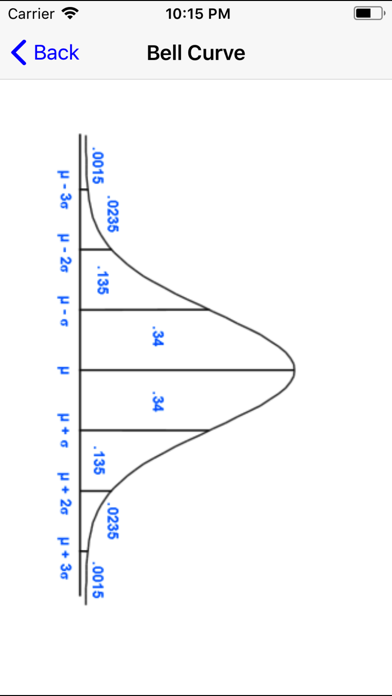Finite Math Pro

iOS UniversalEducation

Free was 0.99

You're taking Finite Mathematics, and you don't have this app? Give yourself an edge!

"Finite Math Pro" contains a rich collection of tutorials, examples, and solvers for the following topics:

Points and Lines:

- Solvers and tutorials to find slopes, intercepts, equations of lines, and graphs of lines
- System solver for 2 variables and 2 equations
- Tutorials for solving systems by graphing, substitution, and linear combinations

Data Analysis:

- Solver to find mean, standard deviation and 5 number summary for up to 30 points
- Tutorial and video lesson

Linear Regression:

- Solver to find the best fit line and correlation coefficient for up to 30 points
- Linear regression tutorial and example

Matrices:

- Adding, subtracting, and multiplying matrices
- Identity matrices
- Solver for 3 variables and 3 equations
- Solvers and tutorials to find determinants/inverses of 2 x 2 and 3 x 3 matrices

Linear Programming (graphical method):

- Tutorial
- Example with step-by-step solution

Probability:

- Formulas
- Survey Results
- Venn Diagrams
- Permutations
- Combinations
- Taking Balls from Urns
- Independent Events
- Games of Chance

Normal Distributions:

- Solve for area under a bell curve without using Z-score tables
- Solve for value on bell curve given area without using Z-score tables
- Examples and tutorials

Compound Interest:

- Solve for amount, principle, time or rate
- Examples, tutorials and video lessons

Please note: there are a lot of solvers, but there aren't solvers for every topic.

I welcome all comments, questions, and suggestions: lfeldman65@gmail.com

Please check out my other applications:

Algebra Pro
ACT Math Prep
Calculus Pro
Geometry Pro
Math Pro (six Math apps for the price of two!)
PreCalculus Pro
Probability Pro
SAT Math Prep
Statistics Pro

What's New

- Formatting enhancements

•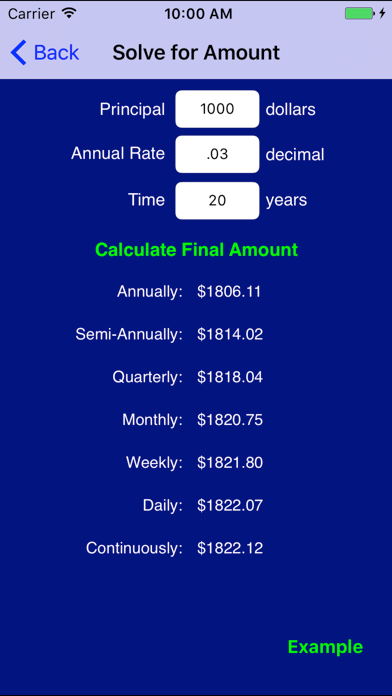•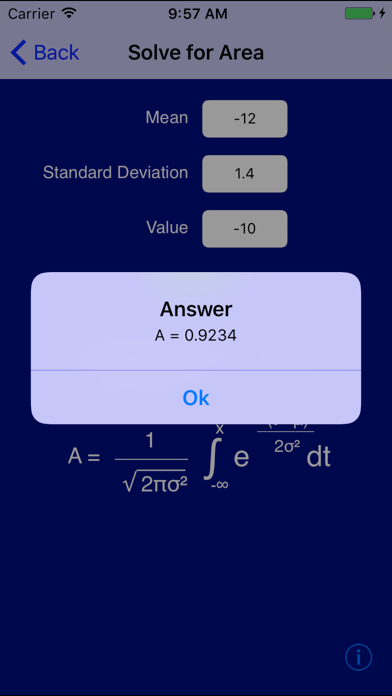•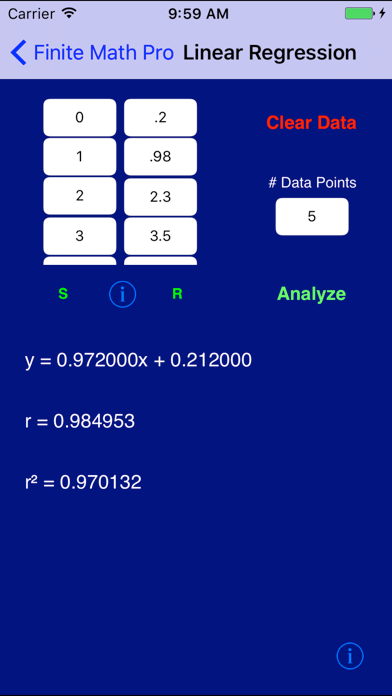•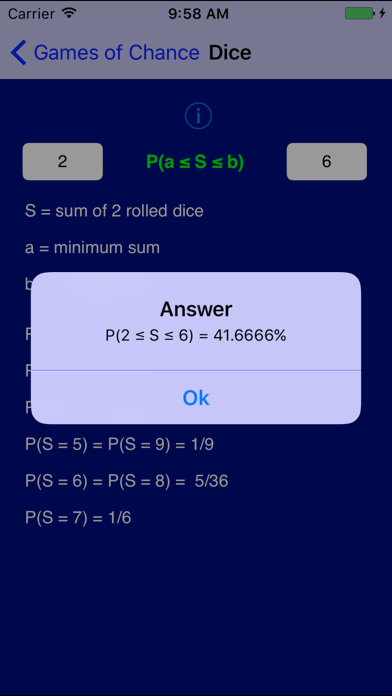•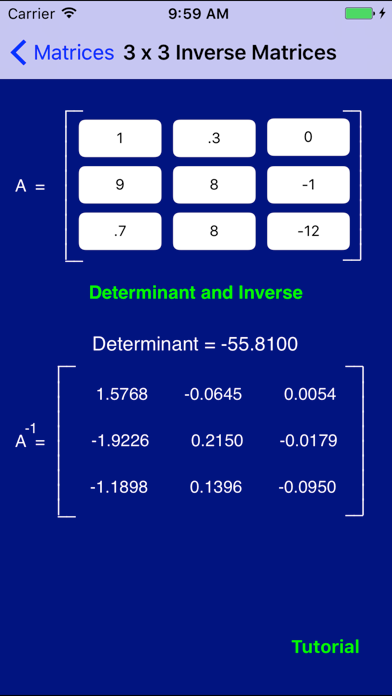•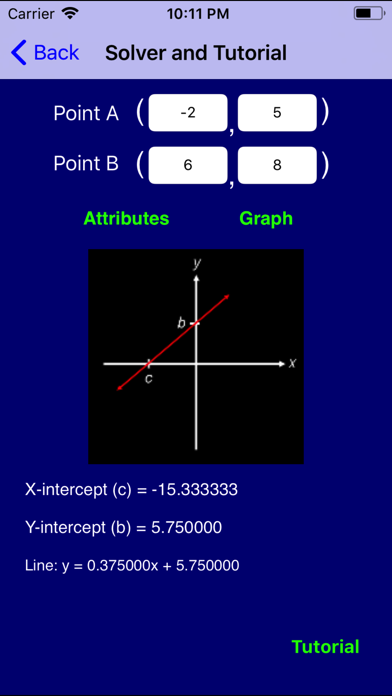•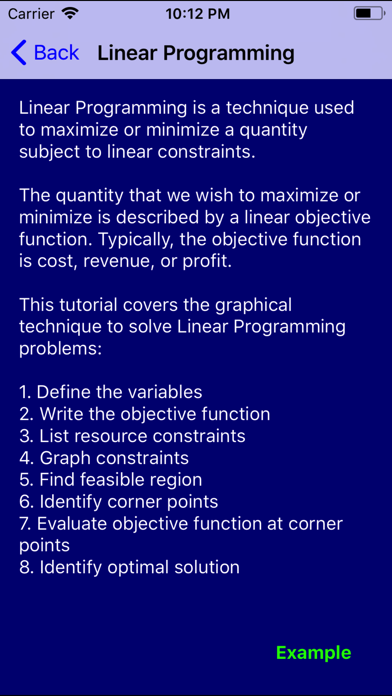•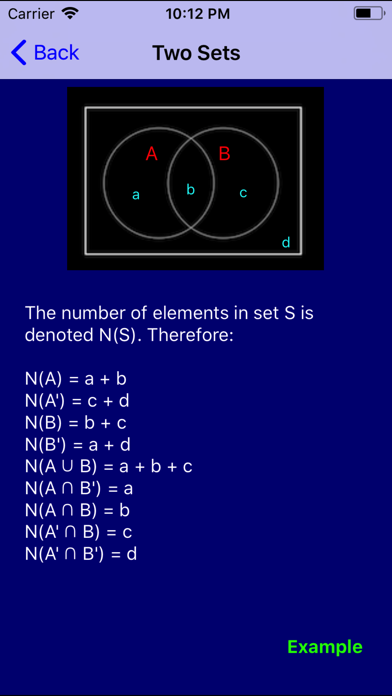••